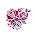## Monday, 17 February 2014

### Minecraft - Music VisualiserNot much to say about this...  Its a music visualiser / graphic equaliser for Minecraft!

I read a post on the raspberry pi forum, by a guy called SpaceGerbil who had created a music visualiser using an 8x8 led matrix, so I went code robbing.

After a pretty major code overhaul, including the removal of a global variable (tish tish), I had a music visualiser for Minecraft, I basically replaced the 8x8 led matrix functions with a class for creating columns of different colour wool in Minecraft.

The only problem was it ran like a dog, a fat asthmatic dog at that, with the music cutting in and out.  So I cranked up the overclocking to the max and increased the chunk size (i.e. the amount of data the program reads ahead and analyses).  This got me to the point where it worked, but the refresh rate was slow, so I moved to 2 Pi's, one running Minecraft, the other running the music analyser and visualiser program, success this was much better!  The video was actually recorded on the PC version of Minecraft using a Bukkit server with the Raspberry Juice plugin with the analyser and visualiser program running on a Pi.

The music visualisation is created by using an FFT algorithm and I am hopeful that I'll be able to change it to use the new interface created which uses the GPU to calculate the FFT's, but the lack of a python interface held me back.  Ill keep on looking though.

You can download the code direct from githubhttps://github.com/martinohanlon/minecraft-music, so run minecraft, open/create a world and follow the instructions:

sudo apt-get install git-core
cd ~
git clone https://github.com/martinohanlon/minecraft-music.git
cd minecraft-music
python minecraft-music.py

Code

```#!/usr/bin/env python

# 8 band Audio equaliser from wav file
# Original code from Space Gerbil
# http://www.raspberrypi.org/phpBB3/viewtopic.php?p=314087

# Heavily Modded to be a minecraft equaliser

import alsaaudio as aa
from struct import unpack
import numpy as np
import wave
import sys
import minecraft
import block
import time
import copy

class MCEqualiser():
def __init__(self):
#open connection to minecraft
self.mc = minecraft.Minecraft.create()
pos = self.mc.player.getTilePos()
#store variables
self.x = pos.x + 5
self.y = pos.y
self.z = pos.z
#clear area
self.drawnMatrix = np.array([0,0,0,0,0,0,0,0])

def drawEqualiser(self, newMatrix):
x,y,z = self.x,self.y,self.z
#loop through the columns
for column in range(0,8):
# only update columns which have changed
if self.drawnMatrix[column] != newMatrix[column]:
# do I need to add or take away block?
if self.drawnMatrix[column] < newMatrix[column]:
self.mc.setBlocks(x+column,y+self.drawnMatrix[column],z,x+column,y+newMatrix[column],z,block.WOOL.id,column)
#  remove blocks
if self.drawnMatrix[column] > newMatrix[column]:
self.mc.setBlocks(x+column,y+self.drawnMatrix[column],z,x+column,y+newMatrix[column],z,block.AIR.id)
self.drawnMatrix = newMatrix.copy()

# Initialise matrix
matrix    = np.array([0,0,0,0,0,0,0,0])
power     = []
weighting = [2,2,8,8,16,32,64,64] # Change these according to taste

# Set up audio
wavfile = wave.open("/home/pi/minecraft-music/hot.wav","r")
sample_rate = wavfile.getframerate()
no_channels = wavfile.getnchannels()
chunk       = 4096
#chunk       = 8192
#chunk       = 16384 #use this value if running it all on one Pi
output = aa.PCM(aa.PCM_PLAYBACK, aa.PCM_NORMAL)
output.setchannels(no_channels)
output.setrate(sample_rate)
output.setformat(aa.PCM_FORMAT_S16_LE)
output.setperiodsize(chunk)

# Return power array index corresponding to a particular frequency
def piff(val):
return int(2*chunk*val/sample_rate)

def calculate_levels(data, chunk,sample_rate,matrix):
#    global matrix
# Convert raw data (ASCII string) to numpy array
data = unpack("%dh"%(len(data)/2),data)
data = np.array(data, dtype='h')
# Apply FFT - real data
fourier=np.fft.rfft(data)
# Remove last element in array to make it the same size as chunk
fourier=np.delete(fourier,len(fourier)-1)
# Find average 'amplitude' for specific frequency ranges in Hz
power = np.abs(fourier)
matrix= int(np.mean(power[piff(0)    :piff(156):1]))
matrix= int(np.mean(power[piff(156)  :piff(313):1]))
matrix= int(np.mean(power[piff(313)  :piff(625):1]))
matrix= int(np.mean(power[piff(625)  :piff(1250):1]))
matrix= int(np.mean(power[piff(1250) :piff(2500):1]))
matrix= int(np.mean(power[piff(2500) :piff(5000):1]))
matrix= int(np.mean(power[piff(5000) :piff(10000):1]))
matrix= int(np.mean(power[piff(10000):piff(20000):1]))
# Tidy up column values for the LED matrix
matrix=np.divide(np.multiply(matrix,weighting),1000000)
# Set floor at 0 and ceiling at 8 for LED matrix
matrix=matrix.clip(0,8)
return matrix

#Create Minecraft Equaliser object
mcequaliser = MCEqualiser()

try:
# Process audio file
print "Processing....."
while data!='':
output.write(data)
matrix=calculate_levels(data, chunk,sample_rate,matrix)
mcequaliser.drawEqualiser(matrix)

except KeyboardInterrupt:
print "User Cancelled (Ctrl C)"

except:
print "Unexpected error - ", sys.exc_info(), sys.exc_info()
raise
```

1.This comment has been removed by the author.

2.This comment has been removed by the author.

3.This comment has been removed by the author.

4.5.6.Really awesome keep up the good work!

7.Really awesome keep up the good work!